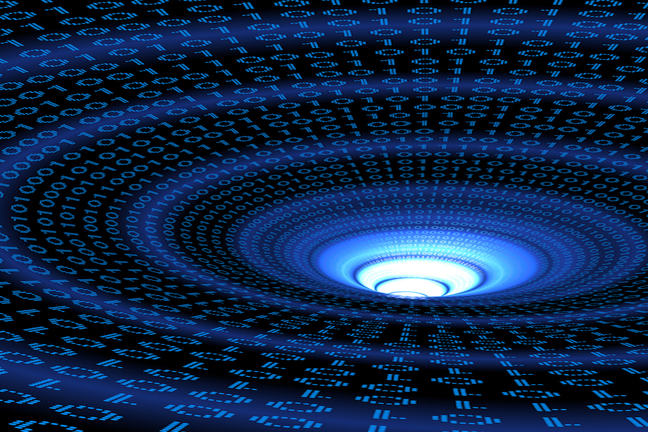2.14

## Weizmann Institute of ScienceBinary codes

# Binary codes

In modern times, once the “digital revolution” came along, there was a need for a new coding system which would be suitable for computers and other electrical-digital devices. The system that was chosen was the binary system, in which all numbers are coded using only the digits 0 and 1. Binary symbology is very important in the computer world. The digits 0 and 1 are called bits. They are translated into electric current flows – the bit 1 symbolises the fact that there is a flow, and the bit 0 symbolises that there is no flow inside the computer. The sequence of these electric symbols is the “language” of the computer, and using it the computer can perform the instructions we give it.

## The binary number system

We write numbers today as ‘strings’ made up of the digits 1,2,3,4,5,6,7,8,9,0. Each digit takes on a different numeric value according to its position. In the number 101, for example, the numeric value of the lefthand 1 is 100, while the numeric value of the righthand 1 is 1. Mathematically speaking, the positional decimal notation that we use determines the value of the number according to powers of ten. Digits written in the units column, the righthand-most digit, retain their numeric value because they are multiplied by 1, which is ten to the power of zero (100). The numerical value of the digits in the next column to the left, the ‘tens’ column, is that digit multiplied by ten to the power of one (101), i.e. 10. and so on. So, the numeric value of the string of digits: 973 is really:
9 x 102 + 7 x 101 + 3 x 100 = 9 x 100 + 7 x 10 + 3 x 1 = 973.

In the binary system, the location of the digits determines their value according to powers of 2. The binary system is a base 2 system, using only the digits 0 and 1. These digits are multiplied by 20=1 when in the column on the extreme right, by 21=2, when in the next column to the left, by 22=4, when in the next column to the left and so on.

Here is the binary table for the first 32 numbers:

Decimal Binary
0 00000
1 00001
2 00010
3 00011
4 00100
5 00101
6 00110
7 00111
8 01000
9 01001
10 01010
11 01011
12 01100
13 01101
14 01110
15 01111
16 10000
17 10001
18 10010
19 10011
20 10100
21 10101
22 10110
23 10111
24 11000
25 11001
26 11010
27 11011
28 11100
29 11101
30 11110
31 11111

## Translating binary to decimal and vice-versa

To translate a binary number into decimal, multiply the rightmost digit by 1 (20), the second digit to the left by 2 (21), the third digit to the left by 4 (22), the fourth digit by 8 (23) and so on. Example: the number 1011 in binary is the decimal 11:
1 x 23 + 0 x 22 + 1 x 21+ 1 x 20 = 1 x 8 + 0 x 4 + 1 x 2 + 1 x 1= 11

There are a few ways to translate a decimal number to binary. The easiest way is to look for the closest power of 2, write a 1 in the corresponding position and subtract from the original number. Continue doing this until you reach zero. Example: the number 36 in binary is: 100100: The closest power of 2 to 36 is 32 which is 25, so we know that the binary number will be 6 digits long with a 1 in the sixth column from the right: 1-----.

36 - 32 = 4 which is 22, so the next ‘1’ bit will be positioned in the third column from the right: 1001--.

4 - 4 = 0, so we have finished and the rest of the bits are zeroes: 100100.